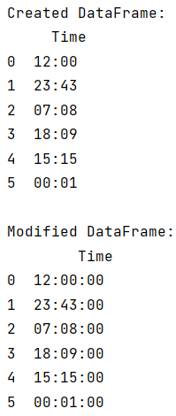# Python Pandas: Convert strings to time without date

Given a Pandas DataFrame, we have to convert strings to time without date.
Submitted by Pranit Sharma, on July 30, 2022

Pandas is a special tool that allows us to perform complex manipulations of data effectively and efficiently. Inside pandas, we mostly deal with a dataset in the form of DataFrame. DataFrames are 2-dimensional data structures in pandas. DataFrames consist of rows, columns, and data.

A string is a group of characters, these characters may consist of all the lower case, upper case, and special characters present on the keyboard of a computer system. A string is a data type and the number of characters in a string is known as the length of the string.

On the other hand, Datetime is a library in python which is a collection of date and time. Inside Datetime, we can access date and time in any format, but usually, date is present in the format of "yy-mm-dd" and time is present in the format of "HH:MM:SS’".

To convert strings to time without date, we will use pandas.to_datetime() method which will help us to convert the type of string. Inside this method, we will pass a particular format of time.

Syntax:

```pandas.to_datetime(
arg,
errors='raise',
dayfirst=False,
yearfirst=False,
utc=None,
format=None,
exact=True,
unit=None,
infer_datetime_format=False,
origin='unix',
cache=True
)
```

Let us understand with the help of an example,

## Python code to convert strings to time without date

```# Import pandas package
import pandas as pd

# import numpy package
import numpy as np

# Creating a dictionary
d = {'Time': ['12:00','23:43','07:08','18:09','15:15','00:01']}

# Creating a Dataframe
df = pd.DataFrame(d)

# Display the dataframe
print('Created DataFrame:\n',df,"\n")

# Converting Time column dtye to time
df['Time'] = pd.to_datetime(df['Time'], format='%H:%M').dt.time

# Display modified DataFrame
print("Modified DataFrame:\n",df)
```

Output: